[BACK]Computer Modeling in Engineering & SciencesDOI: 10.32604/cmes.2022.017708

ARTICLE

Multi-Material and Multiscale Topology Design Optimization of Thermoelastic Lattice Structures

1State Key Laboratory of Structural Analysis for Industrial Equipment, Department of Engineering Mechanics, International Research Center for Computational Mechanics, Dalian University of Technology, Dalian, 116024, China
2Ningbo Research Institute of Dalian University of Technology, Ningbo, 315016, China
3Institute of Structural Health Monitoring and Control, School of Mechanics, Civil Engineering & Architecture, Northwestern Polytechnical University, Xi'an, 710072, China
*Corresponding Author: Zunyi Duan. Email: duanzy@nwpu.edu.cn
Received: 05 June 2021; Accepted: 24 August 2021

Abstract: This study establishes a multiscale and multi-material topology optimization model for thermoelastic lattice structures (TLSs) considering mechanical and thermal loading based on the Extended Multiscale Finite Element Method (EMsFEM). The corresponding multi-material and multiscale mathematical formulation have been established with minimizing strain energy and structural mass as the objective function and constraint, respectively. The Solid Isotropic Material with Penalization (SIMP) interpolation scheme has been adopted to realize micro-scale multi-material selection of truss microstructure. The modified volume preserving Heaviside function (VPHF) is utilized to obtain a clear 0/1 material of truss microstructure. Compared with the classic topology optimization of single-material TLSs, multi-material topology optimization can get a better structural design of the TLS. The effects of temperatures, size factor, and mass fraction on optimization results have been presented and discussed in the numerical examples.

Keywords: Multi-material design optimization; thermoelastic lattice structure; multiscale topology optimization; mass constraint; strain energy

1  Introduction

Analysis and design of thermoelastic structures are an important part of structural design in many mechanical and aerospace engineering applications. Topology optimization  design is an effective strategy to improve the performance of the thermoelastic structure. Many studies had been carried out with considering structure failure caused by thermal stresses . Rodrigues et al.  established a topology optimization model to design the 2D linear thermoelastic structure. Deng et al.  investigated a multi-objective topology design optimization of the thermoelastic structure. Zhang et al. , and Deaton et al.  studied the minimum strain energy and minimum weight design of the thermoelastic structure, respectively. Takalloozadeh et al.  provided a level-set topology optimization model subjected to the thermal loading. Takezawa et al.  optimized the structure considering the structural strength and thermal conductivity constraints.

However, most researchers only focused on single-material topology optimization. For example, Zhang et al. [11,12] studied the single-material topology optimization based on Moving Morphable component method. But the single-material structure design cannot meet the special functional requirements, such as negative thermal expansion structure , zero expansion structure , etc. In order to seek optimal structural performance, the topology design optimization of multi-material lattice structures is proposed. The multi-material structure topology optimization was firstly studied by Thomsen . Then, Sigmund et al.  studied the topology design optimization of three-phase material structures using the SIMP interpolation scheme. By improving the SIMP scheme, the Discrete Material Optimization scheme , Shape Functions with Penalization scheme , and Bi-value Coding Parameterization scheme  were proposed. Multi-material topology optimization considering thermal loads was conducted by Gao et al.  and Giraldo-Londoño et al. . A multiscale topology design optimization with multiple micro materials  was presented. Multi-material topology optimization subjected to multiple volume constraints was investigated by Zhang et al. . López et al.  designed multi-material structures considering structural cost and manufacturability. Ye et al.  proposed an effective method to optimise multi-material structures with the minimum weight as the objective function.

Most of the above research focus on isotropic material, and few types of research consider the multi-material lattice structure. However, the lattice structure is more and more widely used in aerospace and civil engineering because the lattice structure with a variety of porous microstructures [26,27] has the advantages of a high ratio of stiffness . A large number of micro-members in the lattice structure will make the modeling and analysis of the lattice structure difficult. Therefore, multiscale analysis models are utilized to achieve the analysis and optimization of the lattice structure. Zhang et al.  used the EMsFEM to build the analysis model for the lattice structure based on the MsFEM [32,33]. The concurrent multiscale optimization of theTLS subjected to thermal and mechanical loads by using the EMsFEM was provided by Yan et al. [34,35]. Moreover, multiscale topology design optimization of TLSs based on clustering method was proposed by Yan et al. . But there are few studies on multi-material TLSs. By using the same analysis method, the multiscale design optimization of the multi-material TLSs is achieved in the paper. The multi-material and multiscale design optimization of the TLS, where the microstructure is composed of multiple materials, is studied subjected to the thermal and mechanical loading, as shown in Fig. 1. At the macroscale, an artificial element density (Pi\; ) is a design variable to optimize the macroscopic topology configuration of the structure. At the microscale, the cross-sectional (Aj) and material selection parameters (Ej, αj) are design variables to optimize the microscopic unit cell configuration. Considering the manufacturing cost, the unit cell of microscale lattice structural configuration has been adopted.

In the present research, the minimum strain energy of the structure and structural mass are considered as the objective function and constraint, respectively. The paper mainly introduces the following five parts. Section 2 provides the basic formula of the computation of strain energy of the multi-material TLSs by using the EMsFEM. Section 3 elaborates the material interpolation model. The modified SIMP interpolation scheme of the multi-material microstructure is proposed. In Section 4, the problem formulation and sensitivity analysis of the topology optimization of the multi-material TLS are introduced. Section 5 provides numerical examples to achieve multiscale topology design optimization of multi-material TLSs. Conclusions are provided in Section 6.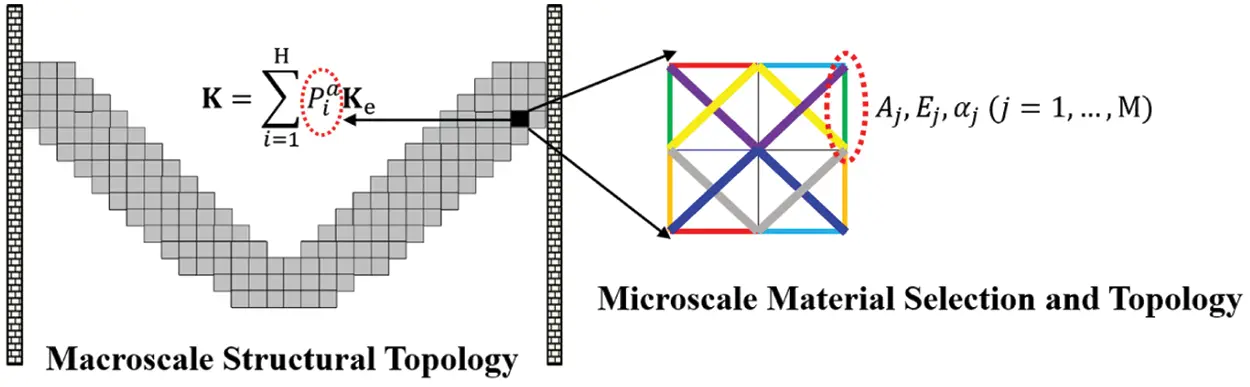Figure 1: Schematic of the multi-material and multiscale topology design optimization of the TLS

2  Strain Energy of Multi-Material TLSs

According to the reference , it indicates that the strain energy of the thermoelastic structure is more useful than the compliance. Thus, the topology design optimization for the TLS with minimum strain energy is studied. This concurrent multiscale optimization for multi-material lattice structure combines the homogenization method with the EMsFEM using the framework of PAMP . The EMsFEM proposed in Zhang et al.  extends the FEM method to multiscale analysis of the structure made of heterogeneous material. In the EMsFEM, the structure is discretized by a number of macro-element and material in macro-element can be heterogeneous. And the stiffness matrix of each macro-element is constructed in a similar way with the traditional FEM but based on numerical shape functions, which is obtained by applying microscale FEM analysis to each macro-element. On the micro-scale, the relationship between the macro-node displacement and micro-node displacement is obtained based on shape functions. On the macro-scale, the displacement of the macrostructure is obtained by traditional FEM analysis methods. As shown in Fig. 2, the multiscale shape function matrix N of a plane four-node element composed of a truss microstructure can be expressed as

N=[Rx(1)TRy(1)TRx(2)TRy(2)TRx(n)TRy(n)T](1)

where n is the number of nodes in the microscopic unit cell. Rx(j) and Ry(j) can be expressed as

{Rx(j)=[N1xx(j)N1xy(j)N2xx(j)N2xy(j)N3xx(j)N3xy(j)N4xx(j)N4xy(j)]Ry(j)=[N1yx(j)N1yy(j)N2yx(j)N2yy(j)N3yx(j)N3yy(j)N4yx(j)N4yy(j)](2)

where Niyx(j) (i=1,\; 2,\; 3,\; 4; j=1,\; 2,,n) is the coupled term of the shape functions. In physical terms, it refers to the nodal displacements of the micro-node j in the truss microstructure in the y-direction, when node i of the macro-element experiences a unit displacement in the x-direction. The specific solution of the shape function with periodic boundary conditions and structural analysis of TLSs based on the EMsFEM can refer to Yan et al. [35,36].

Considering the discrete finite element format, the strain energy of the TLS is presented as

ϕ=12UTKUUTFth+ϕth(3)

where K is the equivalent stiffness matrix (ESM) of the TLS. U is the macro-displacement vector. Fth is the equivalent thermal load vector (ETLV) of the TLS. ϕthis the strain energy with only due to thermal loads.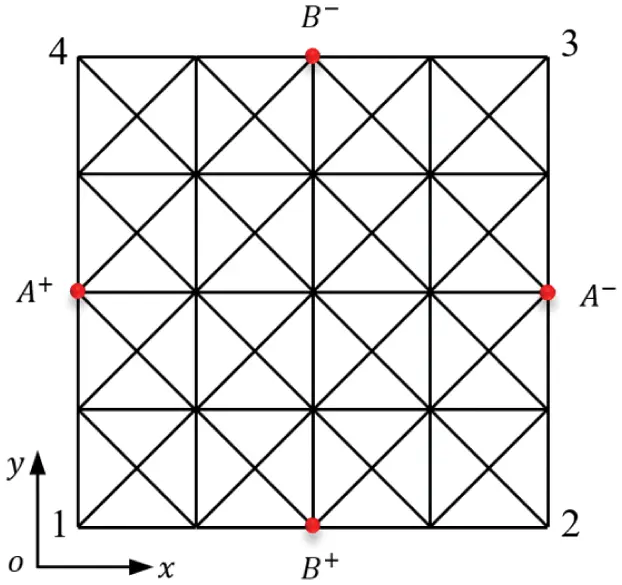Figure 2: The macro-element composed of the truss microstructure

The microstructure consists of multiple materials, as shown in Fig. 1. Each rod is given a candidate material property, and there are M rods with up to M material properties. We can get the whole potential energy of the TLS by assembling the potential energy of all truss unit cells. The ESM and ETLV of the macro-element are determined as

K=i=1HPiaKe=i=1HPia(j=1M(θθjRj)TEjAjLjθθjRj)(4)

Fth=i=1HPiaFeth=i=1HPia(j=1M(θθjRj)TEjAjαjΔT)(5)

ϕth=12i=1Hj=1M(αjΔT)2EjAjLjPia(6)

where H is the number of macro-elements in the structure. M is the number of micro rods in the microstructure. Pi represents the macro-element density. Here a=3 is the penalty coefficient in the method of porous anisotropic materials  to force the macro-density towards 0–1.\;  Ke\; and Feth represent the ESM and ETLV of each macro-element, respectively. Aj and Lj are the cross-sectional area and length of the j-th microrod, respectively. αj is the thermal expansion coefficient of j-th rod. The value range of Aj is [0.0001,0.3]. ΔT denote the temperature rise and Ej is the elastic modulus of j-th rod. The microrod α-β in the two different coordinate systems is shown in Fig. 3. Rj=[Rx({\rm A})TRy({\rm A})TRx({\rm B})TRy({\rm B})T] is the shape function of j-th rod in the truss microstructure.θ=[cosθsinθcosθsinθ] is the coordinate system conversion angle of the rod element. θ is the included angle between the microrod and X axis.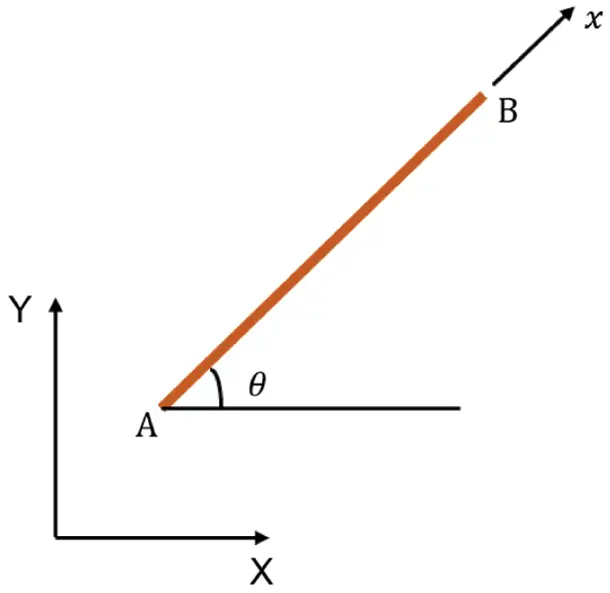Figure 3: A microrod in the two different coordinate systems

3  Material Interpolation Scheme

In order to get a better performance structure, the microstructure is comprised of multiple materials. For a microstructure with n solid materials, the material of each microrob is interpolated by n candidate base materials by using the material interpolation scheme. The SIMP interpolation scheme is modified by the VPHF  to make 0/1 discrete choices clearly. In the optimization problem subjected to mechanical and thermal loading, the elastic modulus and thermal expansion coefficient compute the ESM and ETLV of the macro-element, respectively. The interpolation for elastic modulus\; Ej, thermal expansion coefficient αj and density ρjof the j-th rod be expressed as

Ej=k=1nωkEj(nk+1)(7)

αj=k=1nωkαj(nk+1)(8)

ρj=k=1nωkρj(nk+1)(9)

and

ωk=[1(x¯jkx¯jkδkn)]p=1k1x¯jp(10)

δkn={1,k=n0,kn(11)

where Ej(nk+1) denotes the original elastic modulus of the (nk+1)-th material. αj(nk+1) is the original thermal expansion coefficient of the (nk+1)-th material. ρj(nk+1) is the original density of the (nk+1)-th material.\; x¯jkis the set of design variables obtained by VPHF  according to Eq. (12).

x¯jk={η[eβ(1xjk/η)(1xjk/η)eβ]0xjkηη+(1η)[1eβ(xjkη)1η+(xjkη)eβ1η]η<xjk1(12)

where xjk denotes the design variable of the j-th rod related to the k-th candidate original material.\; β affects the sharpness of the projection. η denotes the parameter obtained by solving the volume conservation equation according to the volume before and after projection. In order to improve calculation efficiency, a constant η=0.5 is adopted here. The curve according to Eq. (12) with different penalty parameters\; β is shown in Fig. 4. As the penalty parameter\; β increases, the penalty effect dramatically increases, which prompts material design variables after the penalty to 0 or 1.Figure 4: Penalty of the VPHF with different\; β values and η=0.5

The elastic modulus, the thermal expansion coefficient and density of the j-th rod in a microstructure interpolated using two materials can express as the following formulations

Ej=x¯j1Ej1+(1x¯j1)Ej2(13)

αj=x¯j1αj1+(1x¯j1)αj2(14)

ρj=x¯j1ρj1+(1x¯j1)ρj2(15)

where x¯j1 denotes the design variable of the j-th rod related to two candidate base materials after projection.

4  Multi-Material and Multiscale Topology Optimization of TLSs

4.1 Optimization Formulation

The topology optimization of the TLS with multi-material microstructure under mechanical and thermal loading is studied. The cross-sectional area of micro rods, macro-elements density, and the candidate base materials of microstructure are design variables. The minimum structural strain energy is chosen as the objective function subjected to the mass constraint. The optimization formulation of multi-material TLS is shown as

Find  Pi,Aj,xjk(i=1,,H)(j=1,,M)(k=1,,n)

Min  Φ=UTKU2UTFth+2ϕth

S.t.  φ=i=1Hj=1MPiAjLjg0ρj/M0φ¯

φk=j=1MAjLjρj(k)/j=1MAjLjρj=φ¯k(k=1,,n)

{ALAjAU0ϵxjk10<ϵPi1(16)

where φk denotes a mass ratio of the k-th candidate base material. The purpose of a given mass ratio constraint for each material in the microstructure is to obtain a multi-material structure. φ¯\; is the upper limit of the mass fraction. g0represents the area of a macro-element. M0 denotes the total mass of the TLS.\; ALand AU\; are upper and lower limits of the cross-sectional area of the microrod, respectively. ϵ denotes lower limit of macro-density to avoid a numerical singularity. φ¯k is the limit of the mass fraction with the k-th candidate base material in microstructure. Other variables share the same meaning with those in Section 2.

4.2 Filter Scheme

To eliminate the checkerboard pattern at the macroscale, a linear density filter [39,40] is utilized. The filtered densityP¯i of element i is expressed as follows

P¯i=xjNieW(xj)vjPjxjNieW(xj)vj(17)

W(xj)=R|xjxi|R(18)

where Nie is the neighborhood of element e. xj is the spatial (center) location of element j. vj is the volume of element j. R is the filter radius of the center of element e.

To reduce the number of gray elements at the macroscale, the VPHF projection is used. Substituting the Eq. (17) into the Eq. (12), the penalized element density P~i= P~i(P¯i(Pj)) can be given.

4.3 Sensitivity Analysis

Sensitivity analysis is mainly divided into the adjoint sensitivity method and direct derivative method. Sensitivity analysis of multi-scale lattice structure can adopt the direct derivative method and the specific process can refer to Yan et al. . The analytical sensitivity of the multi-material TLS can be directly given here. The finite element equilibrium equation of thermoelastic structure is presented as

KU=Fm+Fth(19)

where Fm\; is the applied mechanical load and independent of the design variables. Derivation of the cross-sectional area of the microrod Aj on both sides of the Eq. (19), the derivative of the displacement with respect to the cross-sectional area of the microrod Aj is

UAj=K1Aj(Fm+Fth)+K1FthAj(20)

The derivative of the strain energy with respect to the cross-sectional area of the microrod Aj is

ϕAj=UTKAjU+2UTFthAj2FthK1FthAj2FthK1Aj(Fm+Fth)2UTFthAj+ϕthAj=(2UthU)TKAjU2(Uth)TFthAj+ϕthAj(21)

where Uth denotes the displacement due to thermal loads. The other variables have been defined in Sections 2 and 3. The derivative of the structural ESM, the ETLV, and the strain energy only due to thermal loading with respect to the area of the microrod are shown as

KAj=i=1HP~ia(p=1MRpTAjθθpTkepθθpRp+(θθjRj)TEjLjθθjRj+p=1M(θθpRp)TkepθθpRpAj)(22)

FthAj=i=1HP~ia(p=1MRpTAjθθpT(EpApαpΔT)+(θθjRj)T(EjαjΔT))(23)

ϕthAj=i=1HP~iaEj(αjT)2Lj(24)

Similarly, the sensitivity of micro-scale design variable xjk related to the candidate base materials of microstructure is

ϕxjk=(2UthU)TKxjkU2(Uth)TFthxjk+ϕthxjk(25)

The derivative of the ESM, the ETLV, and the strain energy only due to the thermal loading with respect to the microscopic material design variable xjk are provided

Kxjk=i=1HP~ia(p=1MRpTxjkθθpTkepθθpRp+(θθjRj)TAjLjEjxjkθθjRj+p=1M(θθpRp)TkepθθpRpxjk)(26)

Fthxjk=i=1HP~ia(p=1MRpTxjkθθpT(EpApαpΔT)+(θθjRj)TEjxjk(AjαjΔT)+(θθjRj)Tαjxjk(AjEjΔT))(27)

ϕthxjk=i=1HP~iaLj(Ejxjk(αjT)2+2EjαjT2αjxjk)(28)

where the derivative of the elastic modulus and thermal expansion coefficient with respect to the microscopic material variable are

Ejxjk=Ejx¯jkx¯jkxjk=k=1nωkx¯jkx¯jkxjkEj(nk+1)(29)

αjxjk=αjx¯jkx¯jkxjk=k=1nωkx¯jkx¯jkxjkαj(nk+1)(30)

where the derivative of filtered design variables x¯jkobtained by VPHF  with respect to the microscopic material design variable is

x¯jkxjk={β+eβ(1xjkη)+eβ0xjηβeβ(xjkβ)1η+eβη<xj1(31)

The derivative of the objective function with respect to the macro-density Pi is

ϕPi=P~iPi(aP~ia1(2UthU)TKU2aP~ia1(Uth)TFth+i=1HaP~ia1j=1MEj(αjT)2AjLj)(32)

4.4 Optimization Implementation

The topology optimization flow chart of multi-material TLS is shown in Fig. 5. The specific optimization process is given as follows

(1)   Set the macro-element density, the cross-sectional area of the micro rods, and the number of candidate materials in the microstructure.

(2)   According to the Eq. (17), filter the macro-element density. And penalize the macro-element density and microscopic material design variables by the VPHF  projection.

(3)   According to the multi-material interpolation scheme Eqs. (13)(15) to calculate the elastic modulus matrix E, thermal expansion coefficient matrix α and density matrix ρ.

(4)   According to the Eqs. (4)(6), calculate the structural ESM K, ETLV Fth and strain energy ϕth.

(5)   Solve the equilibrium equation of the multi-material TLS to get the macro-node displacement and get the micro-node displacement through the shape function.

(6)   According to Eqs. (21)(29), calculate structural sensitivity analysis to obtain ϕPi, ϕxjk and ϕAj.

(7)   Optimize the multi-material TLS with the sequential quadratic programming method  and judge the iterative convergence condition. If the program is not convergent, update the design variables and go to Step 2; otherwise, the optimization is finished.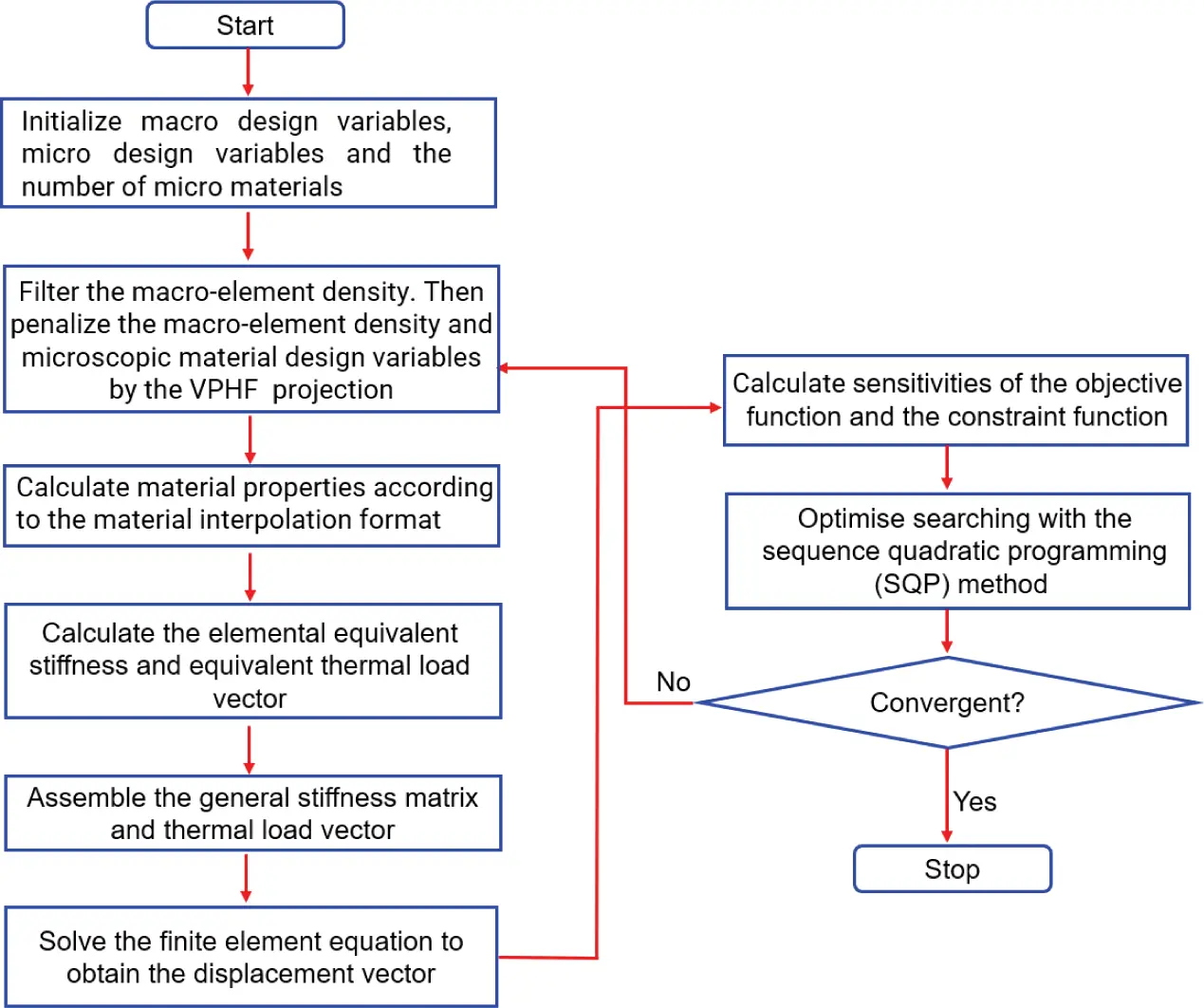Figure 5: Optimization flow chart of the multi-material TLS

5  Numerical Examples

We take the microstructure with two materials as an example. The elastic modulus, thermal expansion coefficients and densities of the two candidate base materials are given in Table 1. Comparing the ratio of elastic modulus to the density of the two materials, it is found that Material 1 is higher than Material 2. The product of the thermal expansion coefficient and elastic modulus is higher than Material 2 compared to Material 1. Thus, Material 2 has the advantage in mechanical properties, and Material 1 has better resistance to thermal deformation. Elastic modulus and thermal expansion coefficients of the microstructure can be obtained by the material interpolation scheme in Section 3, as shown in Fig. 6.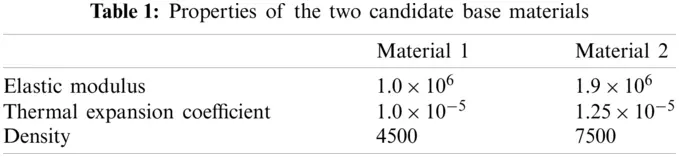In this section, a two-end clamped beam subjected to both mechanical and thermal loading is considered. The length and width of the two-end clamped beam are L = 40 and W = 20, respectively. The mesh is composed of nx×ny=40×20 macroelements, where nx and ny denote the number of macro-elements in the X and Y directions, respectively. Considering the connectivity  between microstructures, a square microstructure consisting of 20 bars is selected, as shown in Fig. 7. In all examples, a mechanical load and a thermal load are applied to the structure as shown in Fig. 7. The mass of the base material available for microunit cell M0=8.39×106 is given. In the optimization formulation of the two-material structure, the mass ratio of materials 1 and 2 are constrained as φ¯1=0.4, φ¯2=0.6. The upper and lower limits of the cross-sectional area of the microrod are 0.0001 and 0.3, respectively. When the cross-sectional area of the microrod reaches a lower limit, the microrod can be ignored due to weak bearing capacity. The above parameters are the same for the following examples.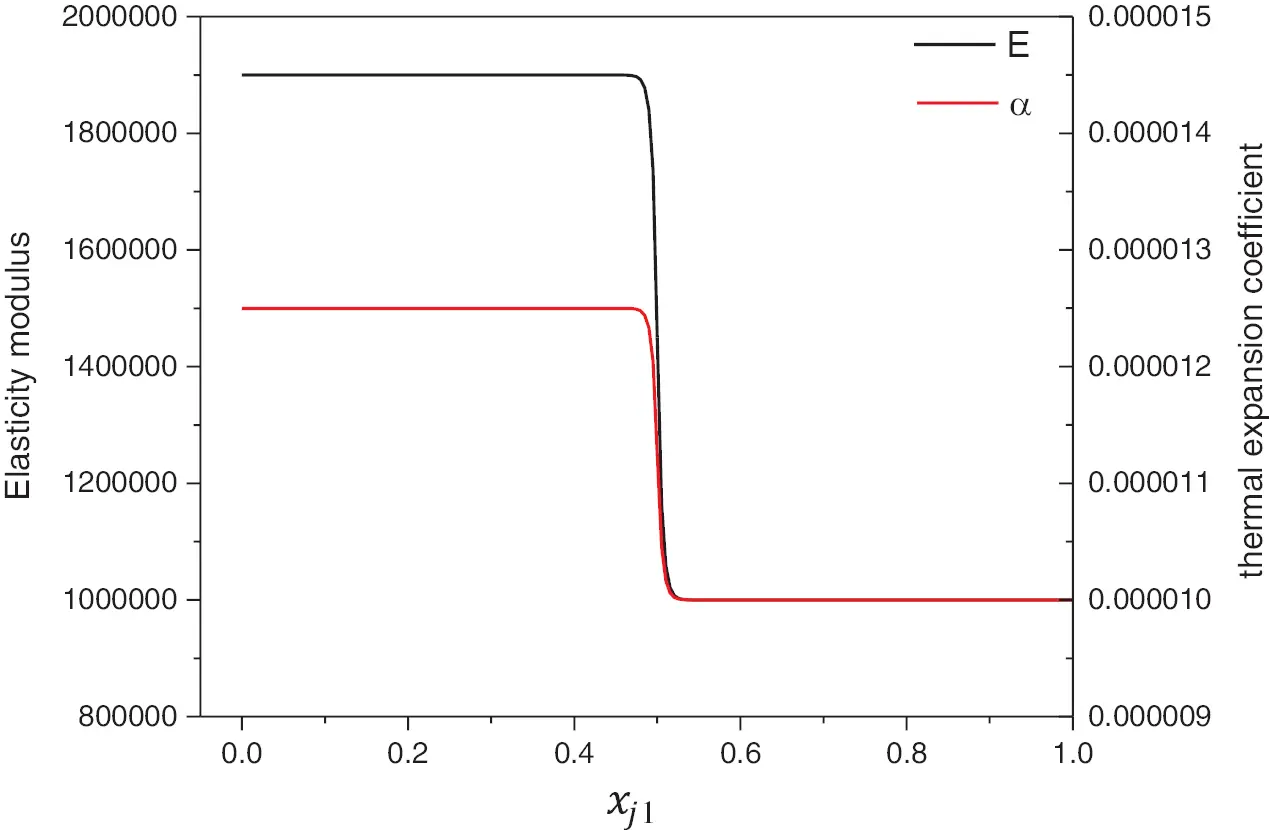Figure 6: Elastic modulus and thermal expansion coefficients of j-th rod of the microstructure based on the material interpolation scheme. (β=100 and η=0.5)

5.1 Comparison of Single-Material Optimization and Multi-Material Optimization

In this example, a mechanical load Fy=1000 and a thermal load ΔT=50°C are applied to the structure. The upper limit of mass fraction is φ¯=0.1. We compare single material optimization results with two material optimization results under the same load conditions and constraints. The minimum strain energy of the multi-material optimization with two materials is 13.50. And the result of the single-material optimization with Material 1 and Material 2 are 15.88 and 14.20, respectively. The mass constraints of all the optimizations are active. Compared with single-material optimization, the strain energy of the optimized multi-material TLS decreases by 15.0% and 4.9%, respectively. We found that the multi-material optimization result is better than the single-material optimization result. As shown in Fig. 8, the macro-configurations of multi-material optimization and single-material optimization are all V-shaped configurations. Comparing the multi-material optimization result with single-material optimization results, we found that more materials are distributed into the microstructure. In the multi-material optimization result, green robs indicate Material 1 and orange robs indicate Material 2. The good mechanical properties are distributed on the X-shaped diagonal in microstructure, used to resist mechanical loads. Multi-material design enhances the bearing properties of microscopic unit cells.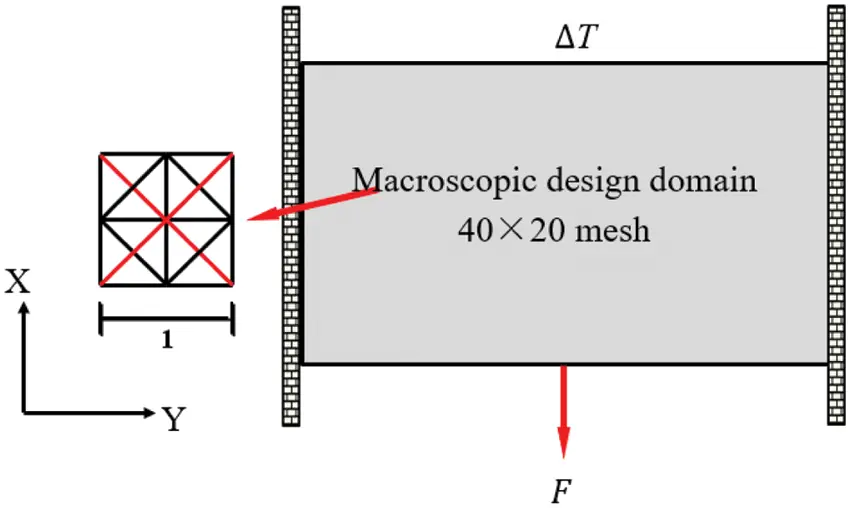Figure 7: Design domain and boundary conditions of a two-end clamped beam

5.2 Multi-Material Optimization with Different Ground Structures Considering Size Factors

In this example, a mechanical load Fy=1000 and a thermal load ΔT=50C are applied to the structure. The upper limit of the mass fraction is φ¯=0.1. Assuming that the microstructure is composed of the same substructures. The length of the vertical rods in the microstructure will change to 1/m of the original length. The size factor m is equal to 1, 2, and 4, as shown in Fig. 9. At the micro-scale, ground structures consist of 6 rods, 20 rods and 72 rods which the size factor m equals 1, 2, and 4. Loads and constraints are the same as mentioned above. The results of the multi-material optimization with the different ground structures are 23.44, 13.50 and 12.99, respectively. The mass constraints are always active. As the number of ground structural members increases, the minimum strain energy of the structure decreases gradually. Because the number of design variables increases, the feasible domain and the possibility of better optimization results become larger. The minimum strain energy at n = 2 is reduced by 42% relative to the minimum strain energy at n = 1. But the minimum strain energy at n = 4 is reduced by 4% relative to the minimum strain energy at n = 2. Therefore, taking the ground structure of n = 2 is the most reasonable, which can reduce the amount of calculation and obtain better optimization results. We obtain the macro-micro topology optimization configuration with different ground structures, as shown in Fig. 10. As the number of ground structural members increases, the amount of material distributed to the macrostructure decreases, and the microstructure material gradually increases. The good mechanical properties are mainly distributed in the diagonal rods of microstructure that is used to resist mechanical loads. The material with good thermal properties distributes in the horizontal rods that can relieve the compressive stress caused by the thermal load in the horizontal direction.Figure 8: Comparison of single-material optimization and multi-material optimization. (a) Macro topological configuration and micro material distribution with the single Material 1 (b) Macro topological configuration and micro material distribution with the single Material 2 (c) Macro topological configuration and micro material distribution with the multi-material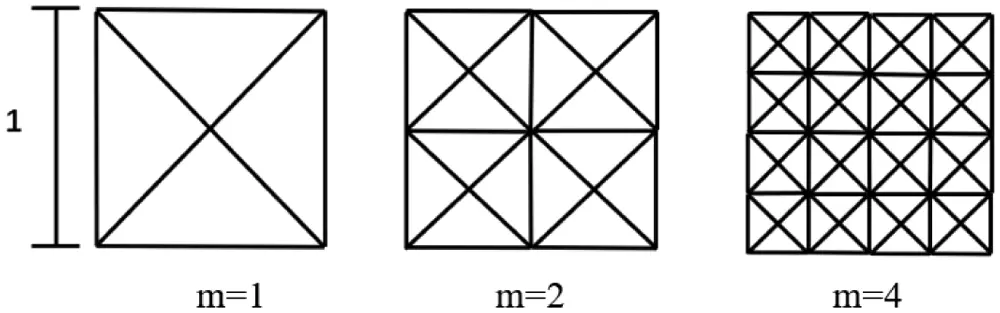Figure 9: Microstructures with different size factors 

5.3 Multi-Material Optimization Results with Different Temperatures

In this example, a mechanical load Fy=1000 is applied to the structure and the upper limit of the mass fraction is φ¯=0.1. The optimization is performed by varying the magnitude of the thermal loads, as shown in Fig. 11. The results of the multi-material TLSs will increase from 12.70, 13.50 to 16.96, when the thermal load ΔT is increased from 0°C, 50°C to 100°C. The mass constraints are always active. The macro topology configurations are all the V-shape with the change of temperatures, but distributions of micro-material will change with the different temperatures, as shown in Fig. 11. And the choice of material properties of each microrod will also change with the different temperature. When there is no temperature load, the horizontal rod with good mechanical Material 2 is mainly subjected to bending. When there is a temperature load, the horizontal rods are distributed with the Material 1 to relieve the compressive stress caused by the thermal loading.

5.4 Multiscale Optimization of the Multi-Material Structure with Different Mass Fractions

In this example, a mechanical load Fy=1000 and a thermal load ΔT=50C are applied to the structure. As shown in Fig. 12, the curve of strain energy of the multi-material TLSs change with the base material mass fraction. As the mass fraction increase, the minimum strain energy gradually decreases, but the downward trend has gradually slowed down. All macro topology configurations develop into V-shape with the change of mass fraction. As the amount of materials increases, the distribution of macroscopic materials firstly will gradually increase, then materials are mainly distributed into the microstructure. The increase of macroscopic materials will increase the mechanical load-bearing capacity of the structure, but at the same time, it will reduce the thermal load-carrying capacity. So it cannot be distributed more in the macrostructure. As the amount of material increases, the material with good mechanical properties is distributed to the longitudinal rod and the diagonal rod at the bottom end, which is used to improve the mechanical bearing capacity and reduce the tensile stress generated by the mechanical load.

In Fig. 13, the curves of the microstructure acturl mass fraction and the macroscopic actural mass fraction change with the constrained mass fraction. The microstructure acturl mass fraction is the ratio of actul microstructure mass to initial microstructure mass. With the increase of the total mass constraint, the microstructure mass fraction gradually increase. Due to the more material distribution into the microstructure, the cross-sectional areas of the micro rods will increase. Comparing the actual total mass fraction with the constrained total mass fraction, we found that the total mass constraint is not active when the constrained mass fraction is larger than 0.50 in this example.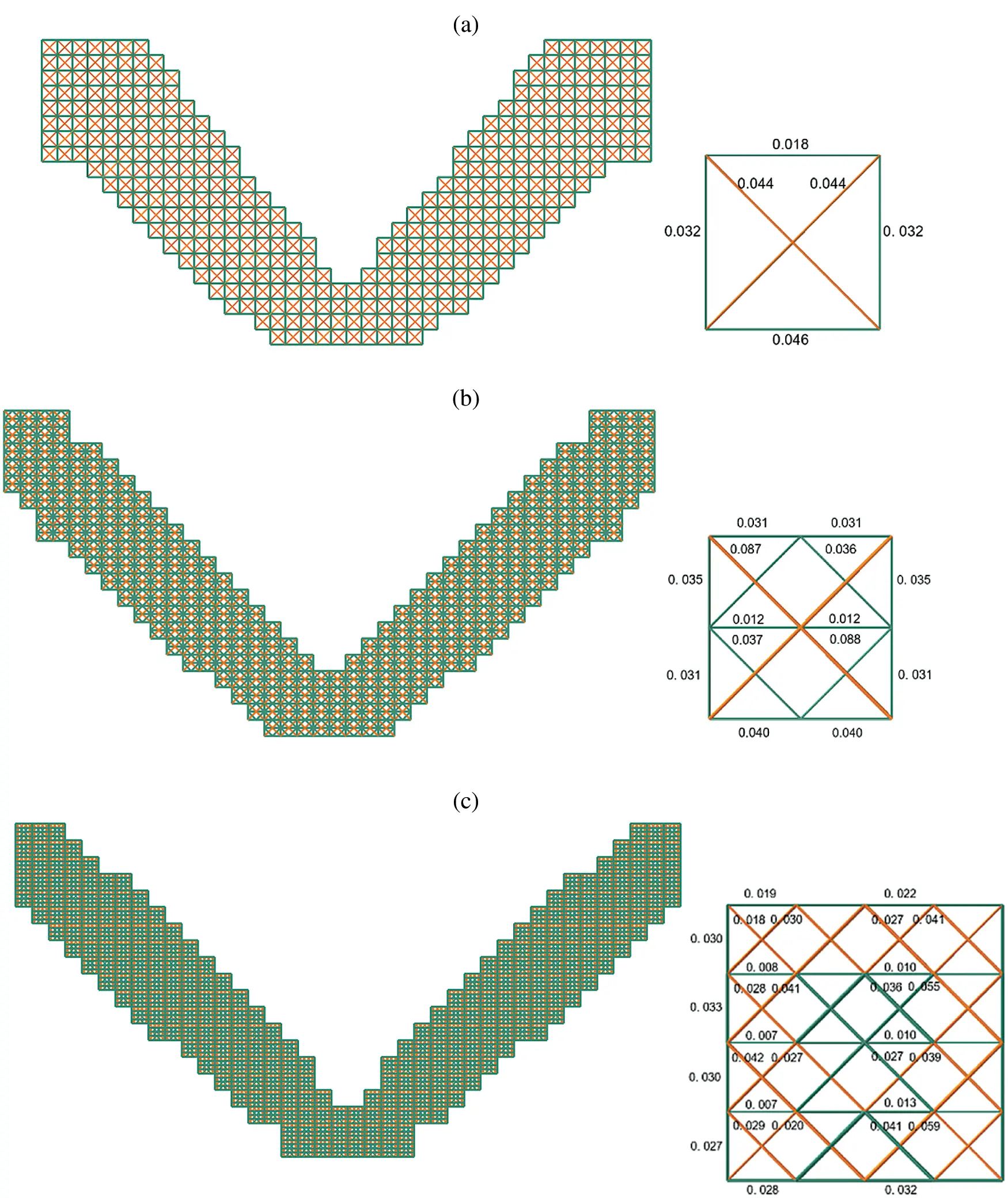Figure 10: Multiscale optimization of multi-material lattice structures with different ground structures. (a) Macro topological configuration and micro material distribution with the size factor m=1 (b) Macro topological configuration and micro material distribution with the size factor m=2 (c) Macro topological configuration and micro material distribution with the size factor m=4Figure 11: Multiscale optimization of multi-material lattice structures with different temperatures. (a) Macro topological configuration and micro material distribution at ΔT=0 (b) Macro topological configuration and micro material distribution at ΔT=50 (c) Macro topological configuration and micro material distribution at ΔT=100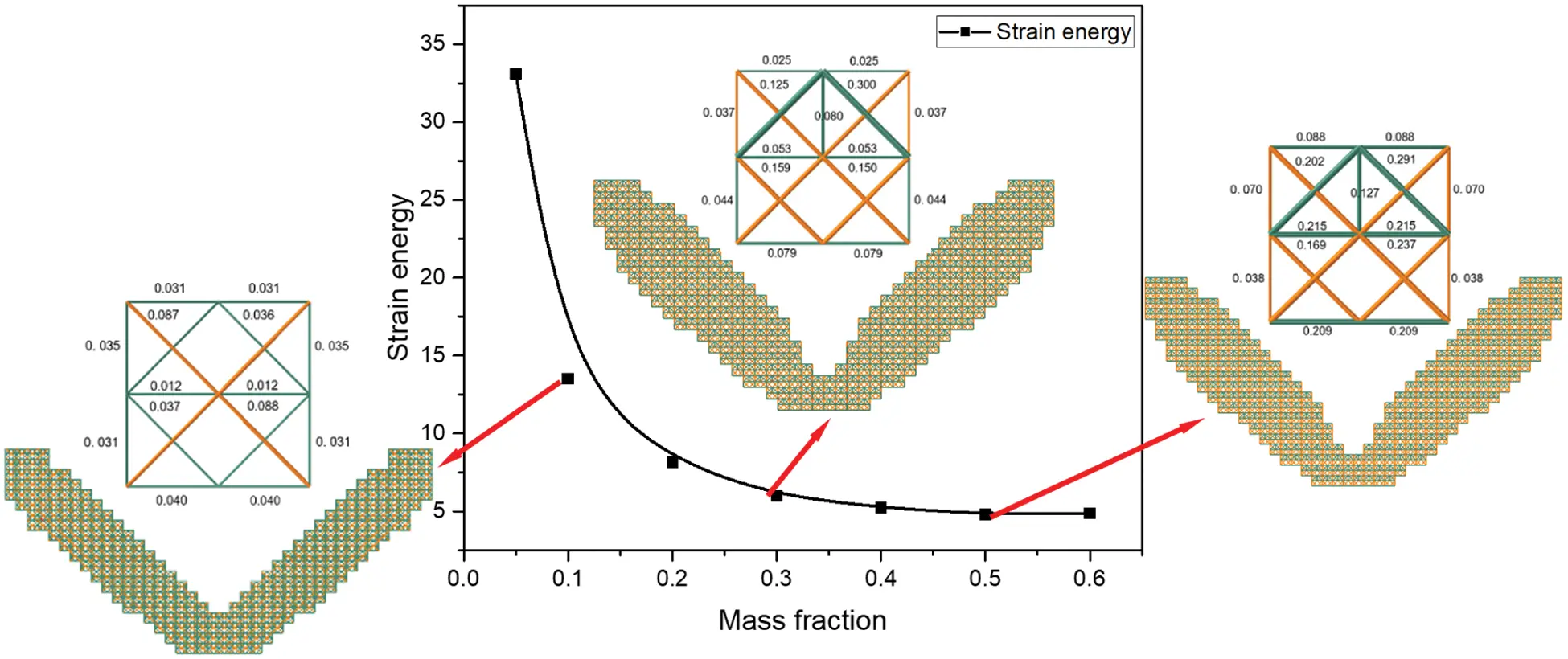Figure 12: Strain energy of the multi-material structure changes with the mass fraction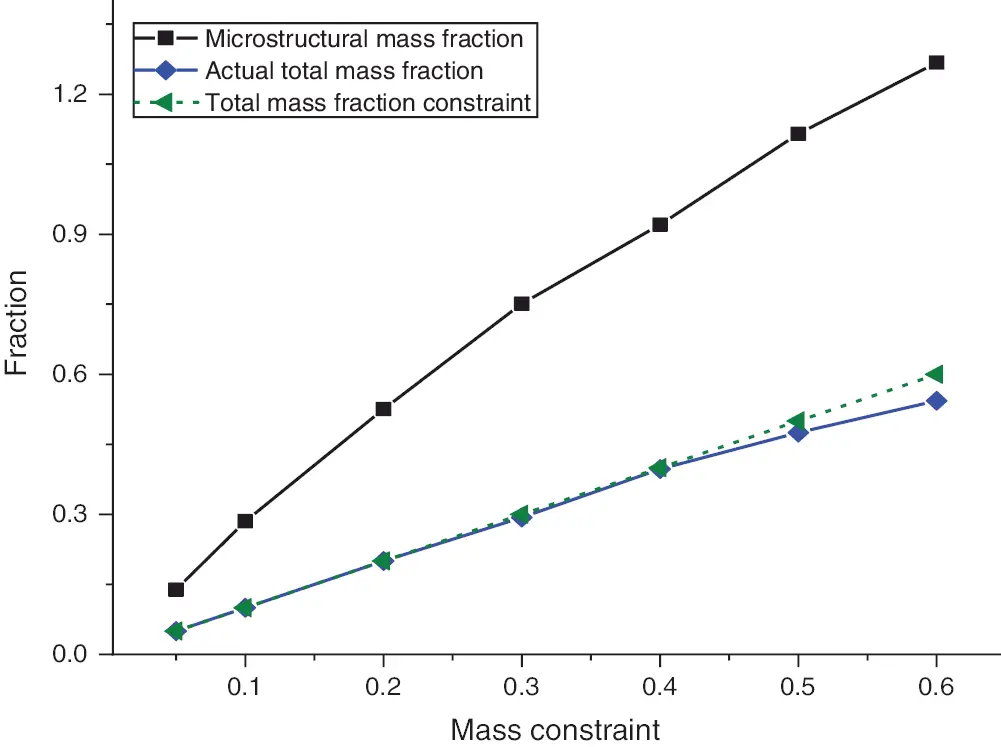Figure 13: Actual material fraction of microscopic and macroscopic structure

6  Conclusion

A multiscale and multi-material topology design optimization model of TLSs subjected to mechanical and thermal loading based on the EMsFEM is established in this research. Different from the traditional minimum compliance optimization, the corresponding multi-material and multiscale mathematical formulation has been established with minimizing strain energy as the objective function. And the mass constraint is considered. The following conclusions are obtained.

The SIMP interpolation scheme has been adopted to realize micro-scale multi-material selection of truss microstructure. The modified VPHF is utilized to obtain a clear 0/1 material of truss microstructure. Compared with the traditional single-material topology optimization, multiscale topology optimization of multi-material TLSs allow increase design freedom for potentially better solutions to obtain the structure for better performance. The influence of temperatures, size factors, and structural mass fraction on the optimization results are introduced. As the temperature and mass fraction increase, the strain energy gradually increases. Temperature and mass fraction have little effect on the macro topological configuration, but have a more significant effect on the distribution of microscopic materials. The macro topological configurations and micro material distributions of multi-material TLSs are presented. The topology optimization of TLS with the microstructure composed of multiple materials realizes the improvement of structural performance.

Funding Statement: This research was financially supported by the National Natural Science Foundation of China (Nos. U1906233, 11732004, Jun Yan; No. 12002278, Zunyi Duan), the Key R&D Program of Shandong Province (2019JZZY010801, Jun Yan), the Fundamental Research Funds for the Central Universities (DUT20ZD213, DUT20LAB308, DUT21ZD209, Jun Yan; G2020KY05308, Zunyi Duan). These supports are gratefully acknowledged. Prof. Jun Yan and Associate Prof. Zunyi Duan received the grants and the URLs to sponsors’ websites are https://www.dlut.edu.cn/, and https://www.nwpu.edu.cn/, respectively.

Conflicts of Interest: The authors declare that they have no conflicts of interest to report regarding the present study.

## References

1. Cheng, K. T., & Olhoff, N. (1981). An investigation concerning optimal design of solid elastic plates. International Journal of Solids and Structures, 17(3), 305-323. [Google Scholar] [CrossRef]
2. Bendsøe, M. P., & Kikuchi, N. (1988). Generating optimal topologies in structural design using a homogenization method. Computer Methods in Applied Mechanics and Engineering, 71(2), 197-224. [Google Scholar] [CrossRef]
3. Allaire, G. (2012). Shape optimization by the homogenization method. USA: Springer-Verlag New York.
4. Sigmund, O. (2001). A 99 line topology optimization code written in matlab. Structural and Multidisciplinary Optimization, 21(2), 120-127. [Google Scholar] [CrossRef]
5. Deaton, J. D., & Grandhi, R. V. (2016). Stress-based design of thermal structures via topology optimization. Structural & Multidisciplinary Optimization, 53, 253-270. [Google Scholar] [CrossRef]
6. Rodrigues, H., & Fernandes, P. (1995). A material based model for topology optimization of thermoelastic structures. International Journal for Numerical Methods in Engineering, 38(12), 1951-1965. [Google Scholar] [CrossRef]
7. Deng, J., Yan, J., & Cheng, G. (2013). Multi-objective concurrent topology optimization of thermoelastic structures composed of homogeneous porous material. Structural and Multidisciplinary Optimization, 47(4), 583-597. [Google Scholar] [CrossRef]
8. Zhang, W., Yang, J., Xu, Y., & Gao, T. (2014). Topology optimization of thermoelastic structures: Mean compliance minimization or elastic strain energy minimization. Structural and Multidisciplinary Optimization, 49(3), 417-429. [Google Scholar] [CrossRef]
9. Takalloozadeh, M., & Yoon, G. H. (2017). Development of pareto topology optimization considering thermal loads. Computer Methods in Applied Mechanics and Engineering, 317, 554-579. [Google Scholar] [CrossRef]
10. Takezawa, A., Yoon, G. H., Jeong, S. H., Kobashi, M., & Kitamura, M. (2014). Structural topology optimization with strength and heat conduction constraints. Computer Methods in Applied Mechanics and Engineering, 276, 341-361. [Google Scholar] [CrossRef]
11. Zhang, W., Jiang, S., Liu, C., Li, D., & Kang, P. (2020). Stress-related topology optimization of shell structures using IGA/TSA-based moving morphable void (MMV) approach. Computer Methods in Applied Mechanics and Engineering, 366, 113036. [Google Scholar] [CrossRef]
12. Zhang, W., Xiao, Z., Liu, C., Mei, Y., & Youn, S. K. (2020). A scaled boundary finite element based explicit topology optimization approach for three-dimensional structures. International Journal for Numerical Methods in Engineering, 121(21), 4878-4900. [Google Scholar] [CrossRef]
13. Takezawa, A., Kobashi, M., & Kitamura, M. (2015). Porous composite with negative thermal expansion obtained by photopolymer additive manufacturing. APL Materials, 3(7), 1917. [Google Scholar] [CrossRef]
14. Lehman, J., & Lakes, R. S. (2014). Stiff, strong, zero thermal expansion lattices via material hierarchy. Composite Structures, 107, 654-663. [Google Scholar] [CrossRef]
15. Thomsen, J. (1992). Topology optimization of structures composed of one or two materials. Structural Optimization, 5(1), 108-115. [Google Scholar] [CrossRef]
16. Sigmund, O., & Torquato, S. (1997). Design of materials with extreme thermal expansion using a three-phase topology optimization method. Journal of the Mechanics and Physics of Solids, 45(6), 1037-1067. [Google Scholar] [CrossRef]
17. Stegmann, J., & Lund, E. (2005). Discrete material optimization of general composite shell structures. International Journal for Numerical Methods in Engineering, 62(14), 2009-2027. [Google Scholar] [CrossRef]
18. Bruyneel, M. (2011). SFP—A new parameterization based on shape functions for optimal material selection: Application to conventional composite plies. Structural & Multidisciplinary Optimization, 43(1), 17-27. [Google Scholar] [CrossRef]
19. Gao, T., Zhang, W., & Pierre, D. (2012). A bi-value coding parameterization scheme for the discrete optimal orientation design of the composite laminate. International Journal for Numerical Methods in Engineering, 91(1), 98-114. [Google Scholar] [CrossRef]
20. Gao, T., Xu, P., & Zhang, W. (2016). Topology optimization of thermo-elastic structures with multiple materials under mass constraint. Computers and Structures, 173, 150-160. [Google Scholar] [CrossRef]
21. Giraldo-Londoño, O., Mirabella, L., Dalloro, L., & Paulino, G. H. (2020). Multi-material thermomechanical topology optimization with applications to additive manufacturing: Design of main composite part and its support structure. Computer Methods in Applied Mechanics and Engineering, 363, 112812. [Google Scholar] [CrossRef]
22. Xu, L., & Cheng, G. (2018). Two-scale concurrent topology optimization with multiple micro materials based on principal stress orientation. Structural & Multidisciplinary Optimization, 57(5), 2093-2107. [Google Scholar] [CrossRef]
23. Zhang, X. S., Paulino, G. H., & Ramos, A. S. (2018). Multi-material topology optimization with multiple volume constraints: A general approach applied to ground structures with material nonlinearity. Structural and Multidiplinary Optimization, 57, 161-182. [Google Scholar] [CrossRef]
24. López, C., Burggraeve, S., Lietaert, P., Stroobants, J., & Desmet, W. (2020). Model-based, multi-material topology optimization taking into account cost and manufacturability. Structural and Multidisciplinary Optimization, 62(1), 2951-2973. [Google Scholar] [CrossRef]
25. Ye, H. L., Dai, Z. J., Wang, W. W., & Sui, Y. K. (2019). ICM method for topology optimization of multimaterial continuum structure with displacement constraint. Acta Mechanica Sinica, 35(3), 552-562. [Google Scholar] [CrossRef]
26. Evans, A. G., Hutchinson, J. W., Fleck, N. A., Ashby, M., & Wadley, H. (2001). The topological design of multifunctional cellular metals. Progress in Materials Science, 46(3–4), 309-327. [Google Scholar] [CrossRef]
27. Yi, B., Zhou, Y., Yoon, G. H., & Saitou, K. (2019). Topology optimization of functionally-graded lattice structures with buckling constraints. Computer Methods in Applied Mechanics and Engineering, 354(SEP.1), 593-619. [Google Scholar] [CrossRef]
28. Zhang, X., Vyatskikh, A., Gao, H., Greer, J. R., & Li, X. (2019). Lightweight, flaw-tolerant, and ultra strong nano architected carbon. Proceedings of the National Academy of Sciences, 116(14), 201817309. [Google Scholar] [CrossRef]
29. Hou, S., Li, Q., Long, S., Yang, X., & Wei, L. (2008). Multiobjective optimization of multi-cell sections for the crashworthiness design. International Journal of Impact Engineering, 35(11), 1355-1367. [Google Scholar] [CrossRef]
30. Zhang, Y., Xiao, M., Li, H., Gao, L., & Chu, S. (2018). Multiscale concurrent topology optimization for cellular structures with multiple microstructures based on ordered SIMP interpolation. Computational Materials Science, 155, 74-91. [Google Scholar] [CrossRef]
31. Zhang, H., Lv, J., & Zheng, Y. (2010). A new multiscale computational method for mechanical analysis of closed liquid cell materials. Computer Modeling in Engineering & Sciences, 68(1), 55. [Google Scholar] [CrossRef]
32. Hou, T. Y., & Wu, X. H. (1997). A multiscale finite element method for elliptic problems in composite materials and porous media. Journal of Computational Physics, 134(1), 169-189. [Google Scholar] [CrossRef]
33. Efendiev, Y., & Hou, T. (2007). Multiscale finite element methods for porous media flows and their applications. Applied Numerical Mathematics, 57(5), 577-596. [Google Scholar] [CrossRef]
34. Yan, J., Hu, W., & Duan, Z. (2015). Structure/material concurrent optimization of lattice materials based on extended multiscale finite element method. International Journal for Multiscale Computational Engineering, 13(1), 73-90. [Google Scholar] [CrossRef]
35. Yan, J., Yang, S., & Yang, C. (2014). Multiscale analysis of thermal stress of lattice materials and its size effects. Journal of Thermal Stresses, 37(8), 885-904. [Google Scholar] [CrossRef]
36. Yan, J., Sui, Q., Fan, Z., Duan, Z., & Yu, T. (2020). Clustering-based multiscale topology optimization of thermo-elastic lattice structures. Computational Mechanics, 66, 979-1002. [Google Scholar] [CrossRef]
37. Liu, L., Yan, J., & Cheng, G. (2008). Optimum structure with homogeneous optimum truss-like material. Computers & Structures, 86(13), 1417-1425. [Google Scholar] [CrossRef]
38. Xu, S., Cai, Y., & Cheng, G. (2010). Volume preserving nonlinear density filter based on heaviside functions. Structural & Multidisciplinary Optimization, 41(4), 495-505. [Google Scholar] [CrossRef]
39. Bourdin, B. (2001). Filters in topology optimization. International Journal for Numerical Methods in Engineering, 50(9), 2143-2158. [Google Scholar] [CrossRef]
40. Borrvall, T. (2001). Topology optimization of elastic continua using restriction. Archives of Computational Methods in Engineering, 8(4), 351-385. [Google Scholar] [CrossRef]
41. Boggs, P. T., & Tolle, J. W. (1995). Sequential quadratic programming. Acta Numerica, 4, 1-51. [Google Scholar] [CrossRef]
42. Du, Z., Zhou, X. Y., Picelli, R., & Kim, H. A. (2018). Connecting microstructures for multiscale topology optimization with connectivity index constraints. Journal of Mechanical Design, 140(11), 111417. [Google Scholar] [CrossRef]This work is licensed under a Creative Commons Attribution 4.0 International License, which permits unrestricted use, distribution, and reproduction in any medium, provided the original work is properly cited.